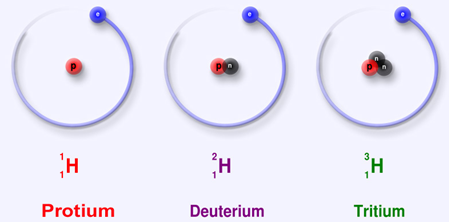> > Atomic Mass and Molecular Mass

# Atomic Mass and Molecular Mass

You fairly have a rough idea of what atoms and molecules are. But, did you ever wonder that atomic mass and molecular mass are important concepts as well? What do we do by knowing the mass of such tiny atoms and molecules? Well, the significance of these atomic and molecular masses is huge! In this chapter, we will cover the concepts and also see how important they are.

### Suggested VideosAtomic MassDalton's Atomic TheoryStoichiometry## Atomic Mass

An atom is such small a particle that it cannot be seen or isolated. Therefore, it is impossible to determine the actual mass of a single atom by weighing it. However, Avogadro’s hypothesis finally solved the problem. He took equal volumes of two different gases under similar conditions of temperature and pressure. He then weighed them.

Surprisingly, the ratio of their masses was equal to the ratio of their single molecules. Thus, though the actual masses of the atoms could not be determined, their relative masses could be determined. If the atomic mass of hydrogen is taken as 1, the relative atomic mass of oxygen is 16.

Initially, scientists obtained the atomic masses of all the elements by comparing with the mass of hydrogen taken as 1. However, the problem was that the atomic masses of most of the elements came out to be fractional in this method. Therefore, carbon is taken as the reference for the determination of atomic masses.

### Browse more Topics under Some Basic Concepts Of Chemistry

Learn about Stoichiometry and Stoichiometric Calculations here.

## What is Atomic Mass?

Atomic mass of an element is the number of times an atom of that element is heavier than an atom of carbon taken as 12. One atomic mass unit is equal to one-twelfth of the mass of an atom of carbon 12 isotope. The atomic mass of an element is the average relative mass of its atoms as compared to an atom of carbon 12 taken as 12.

Fractional abundance of an isotope is the fraction of the total number of atoms that is comprised of that particular isotope. Atomic mass of an element = (Fractional abundance of isotope 1 × mass of isotope 1) + (Fractional abundance of isotope 2 × mass of isotope 2)### Gram Atomic Masses

The atomic masses of elements expressed in grams is their gram atomic masses. For eg: the atomic mass of oxygen is 16 amu.

∴ Gram atomic mass of oxygen = 16 g.

## Molecular Mass

The molecular mass of a substance is the number of times the molecule of the substance is heavier than one-twelth the mass of an atom of carbon -12. Or, the molecular mass is equal to the sum of its atomic masses of all the atoms present in one molecule of a substance. For eg: Water (H2O)

Atomic mass of H= 1 unit
Atomic mass of O =16 units
The molecular mass of water = 2 × atomic mass of H+1 × atomic mass of O
= 2 × 1 + 16 × 1
= 18 units

### Gram Molecular Mass

The molecular mass of a substance expressed in grams is the gram molecular massFor eg: Molecular mass of oxygen = 32u
∴ Gram molecular mass of oxygen = 32 g

Learn how to calculate the number of mole and equivalent weight.

## Solved Example For You

Q: The specific heat of a metal of atomic mass 32 is likely to be:

1. 0.25
2. 0.24
3. 0.20
4. 0.15

Solution: Specific heat = 6.4/atomic mass

Therefore, Specific Heat = 6.4/32 = 0.2

Share with friends

## Customize your course in 30 seconds

##### Which class are you in?
5th
6th
7th
8th
9th
10th
11th
12th
Get ready for all-new Live Classes!
Now learn Live with India's best teachers. Join courses with the best schedule and enjoy fun and interactive classes.Ashhar Firdausi
IIT Roorkee
BiologyDr. Nazma Shaik
VTU
ChemistryGaurav Tiwari
APJAKTU
Physics
Get Started

## Browse

##### Some Basic Concepts of Chemistry0 Followers

Most reacted comment
1 Comment authorsRecent comment authors
Subscribe
Notify ofGuest

Where are Equivalent weight video in chapter

## Question Mark?

Have a doubt at 3 am? Our experts are available 24x7. Connect with a tutor instantly and get your concepts cleared in less than 3 steps.